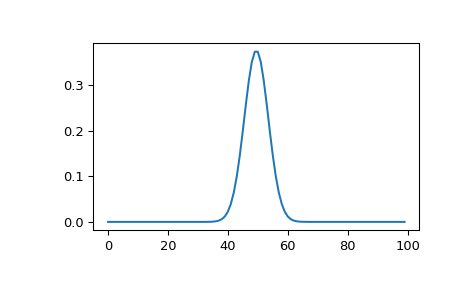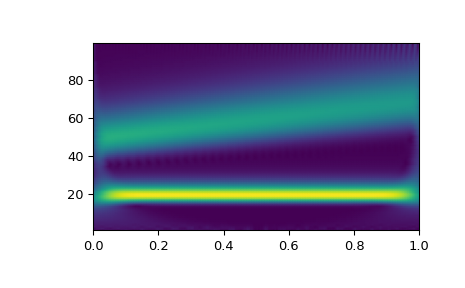# scipy.signal.morlet2¶

scipy.signal.morlet2(M, s, w=5)[source]

Complex Morlet wavelet, designed to work with cwt.

Returns the complete version of morlet wavelet, normalised according to s:

exp(1j*w*x/s) * exp(-0.5*(x/s)**2) * pi**(-0.25) * sqrt(1/s)

Parameters
Mint

Length of the wavelet.

sfloat

Width parameter of the wavelet.

wfloat, optional

Omega0. Default is 5

Returns
morlet(M,) ndarray

morlet

Implementation of Morlet wavelet, incompatible with cwt

Notes

New in version 1.4.0.

This function was designed to work with cwt. Because morlet2 returns an array of complex numbers, the dtype argument of cwt should be set to complex128 for best results.

Note the difference in implementation with morlet. The fundamental frequency of this wavelet in Hz is given by:

f = w*fs / (2*s*np.pi)


where fs is the sampling rate and s is the wavelet width parameter. Similarly we can get the wavelet width parameter at f:

s = w*fs / (2*f*np.pi)


Examples

>>> from scipy import signal
>>> import matplotlib.pyplot as plt

>>> M = 100
>>> s = 4.0
>>> w = 2.0
>>> wavelet = signal.morlet2(M, s, w)
>>> plt.plot(abs(wavelet))
>>> plt.show()This example shows basic use of morlet2 with cwt in time-frequency analysis:

>>> from scipy import signal
>>> import matplotlib.pyplot as plt
>>> t, dt = np.linspace(0, 1, 200, retstep=True)
>>> fs = 1/dt
>>> w = 6.
>>> sig = np.cos(2*np.pi*(50 + 10*t)*t) + np.sin(40*np.pi*t)
>>> freq = np.linspace(1, fs/2, 100)
>>> widths = w*fs / (2*freq*np.pi)
>>> cwtm = signal.cwt(sig, signal.morlet2, widths, w=w)
>>> plt.pcolormesh(t, freq, np.abs(cwtm), cmap='viridis', shading='gouraud')
>>> plt.show()#### Previous topic

scipy.signal.ricker

scipy.signal.cwt